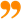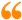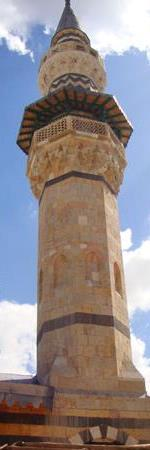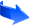## The Original Days of Event

The Original Days of Event:
We have explained in sections II.15-16 that there is a minimum 'Day' which is that indivisible duration of time called 'the Single Day' (al-yawm al-fard). And we showed that this Day—when taken globally, or indeed universally (since it encompasses all of manifest creation)—actually includes our normal day, though we encounter it in fact just as a moment. In each one of these moments or Days there is only one global cosmic 'event' happening in each part of the entire cosmos. These Single Days are the original cosmic Days which are called the divine 'Days of event' (ayyâm-ul-sha’n), as discussed in the preceding Chapter and Chapter II. For in each Day of event (or divine 'task') Allah creates a single event actually encompassing every entity and phenomenon of the whole manifest world. As He said in the Qur’an: each Day He is upon a (single) task (55:29).
Ibn ‘Arabî points out that 'in each day of our normal days, that is from sunset to sunset or from sunrise to sunrise, there is the end of three hundred and sixty days…' [Ayyâm Al-Sha’n: 6] (assuming the circle is 360 degrees). That is because in every moment of the normal day that there is the end (or the beginning) of a day in one place, there is a corresponding beginning (or end) of another day in another place.
To explain this further, let us divide the circumference of the earth into 360 longitudinal lines and the day into 360 degrees of longitude. Therefore, any whole day that we encounter in any specific place is a combination or the sum of the ends (last degrees) of 360 days from other places on the earth; or in the same way, it is the sum of the first degrees of 360 days around the earth. For example let us suppose that we are on the first longitudinal line at the first degree of the day, then the second degree of this day on this first longitudinal line is the first degree of the day beginning on the second line, and so on. In another way we can also say that the 360 degrees of the 'day' on the first line are the collection of the last degrees of the lines 360, 359, 358 and so on up to line number 1. Therefore in every moment there is one full day around the earth: now, for example, it is morning somewhere, noon somewhere else and evening and midnight in other places; but all in total is a single full day (see also section II.15 above).
So the flow of these original Days marks the actual sequence of events that spans space and time. But because we live in and can only observe a tiny point of the whole space of the globe, we encounter linear time as our normal observed days of the week (i.e. the 'circulated days' described in the next section). Therefore, these original 'Days of event' are intertwined with our normal observed days.

============
 Mathematically we can divide the circle into 360 degrees, 400 grads, 2p radians or indeed to any number of units. Ibn ‘Arabî, however, affirms that the 360-degree system has a divine origin, which is the total number of prime divine forms of knowing (‘ilm) that the Universal Intellect (the 'Higher Pen') was taught by the 'Greatest Element' (Nûn) [I.295.8, alluding to the standard cosmological interpretation of the Qur’anic symbols at 68:1]. Also in Al-Tanazzulât Al-Layliyya fî Al-Ahkâm Al-Ilâhiyya, he mentioned on page 35 that the Intellect has 360 faces towards the divine Presence (Al-Hadra Al-Ilâhiyya).

System.String[]إنَّ الذكر من أنفع العبادات وأعظمها، والغفلة عنه خطأ كبير، وخطر على قلب العبد، فليحذر المسلم من ترك الذكر وليرطب لسانه بذكر الله في كلِّ وقت، وفي كلِّ مكان، حتى إذا جاءت لحظة الفراق فإذا هو ينطق بـ (لا إله إلا الله) وتكون آخر كلامه، فهنيئاً لمن وفَّقه الله وختم له بخاتمة السعداء.فضيلة الشيخ الدكتور رمضان صبحي ديب الدمشقي [الفصل السابع من كتاب إيجاز البيان عن سيرة فضيلة الشيخ رمضان - -]## مشاركة الصفحةالإعجاب بصفحتنا على الفيسوك:الإعجاب بصفحتنا على الفيسوك: